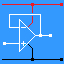RANDOM PAGE

SITE SEARCH

LOG
IN

HELP

# Writing Specifications

This is the AQA version closing after June 2019. Visit the the version for Eduqas instead.

## To Pass Your Coursework, You Must Measure Electronic Values

Here are some examples of subsystem specifications. Some of these might be applicable to your project.

A useful specification that gets project marks must contain measurable values such as ...

• Voltage - measured with a multimeter
• Current - measured with a multimeter
• Power - measure voltage with a multimeter and calculate the power using V2 / R
• Frequency - measured with an oscilloscope
• Time - measured with an oscilloscope / stop watch / clock
• Temperature - measured with a thermometer
• Light Level - measured with a Lux Meter
• Sound level - measured with a sound level meter.

## Example Specifications

Use a table with numbered rows. These row numbers can be referred to later in your report.

Measurable Parameter Reasons for Choice How it will be tested What test equipment to use
Give a range of values or a value +/- 5% for example. Reasons will based on research from the data sheets or from practical investigations. Explain how the parameter will be tested or calculated. Name the test equipment to be used.

### 1) LED

LED Current will be 5 to 10 mA The data sheet specifies 20mA, absolute maximum. Use Ohm's law to calculate a safe series resistor. Use a multimeter to confirm the current is within the range.

### 2) LM386 Voltage

Run on 5 to 15 Volts This voltage range is specified in the LM386 data sheet. Test the 5 and 15 V extremes and several intermediate voltages. Use a multimeter.

### 3) LM386 Power

Produce at least 250mW. The data sheet indicates that this is possible. Measure the audio output voltage and calculate Vrms2 / R Use an oscilloscope.

### 4) LM386 dB Level

Produce at least 60 dB sound level one metre from the loudspeaker. In my first practical investigation, I measured the sound level in a quiet room at 50dB. To hear the amplifier in a noisier room, the output must be more than 60dB. Measure the dB level one metre away from the loudspeaker. Use a sound level meter.

### 5) 555 Astable Voltage

4.5 to 16 Volts These values are specified in the data sheet. As the circuit is to run on a car battery, the 12 to 15 volt range will be tested. Use an adjustable power supply and a multimeter.

### 6) 555 Astable Frequency

3500 to 3800 Hz In my second practical investigation, I connected a sounder to a signal generator and found out the this range of frequencies sounded a lot louder than other frequencies. Measure the period using an oscilloscope and calculate the frequency OR use a frequency meter. Oscilloscope or Frequency Meter.

### 7) 555 Astable Loudness

More than 60 dB. In my first practical investigation, I measured the sound level in a quiet room at 50dB. To hear the buzzer in a noisier room, the output must be more than 60dB. Measure the dB level one metre away from the loudspeaker. Use a sound level meter.

## Measurement Accuracy - For A2

High scoring A2 projects must include information about the accuracy of all measurements.

1. Meters with a linear scale can be read to +/- the smallest division.
1. A ruler is accurate to +/- 1mm.
2. A thermometer might be accurate to +/- one degree (temperature).
3. A protractor might be accurate to +/- one degree (angle).
2. Low cost multimeters, when new, are accurate to about +/- 1%. After student abuse, they can deteriorate to +/- 10%. We throw away meters worse than this.
3. Check your multimeter by comparing it with a couple of similar or better multimeters.
4. Meters with a digital display can, at best, be accurate to +/- the last digit.
If the meter reads 2.75 Volts that will be +/- 0.01 Volts. It the meter is accurate to 1%, a reading of 2.75 Volts will be about +/- 0.3 Volts.
5. Conventional oscilloscopes can be read to +/- a small division on the screen. This is not very accurate. You'd be lucky if your reading was accurate to +/- 2%. If the wave fills only half the vertical screen area, the accuracy drops to +/- 4%. Remember to set the oscilloscope controls to the "Cal" position. If you forget, your measurements will simply be wrong!
6. Digital oscilloscopes are limited by the resolution of their A to D converter. If it has an 8 bit converter, the input is divided up into 28 levels so the reading is, at best, accurate to +/- 1/256th of the full scale reading.
7. It is particularly hard to estimate the accuracy of meters with logarithmic scales. These include Lux and Sound level meters. Estimate the uncertainty by looking at the scale and seeing the next higher or lower reading you could sensibly take. This is roughly the size of your uncertainty.

reviseOmatic V3     Contacts, ©, Cookies, Data Protection and Disclaimers Hosted at linode.com, London